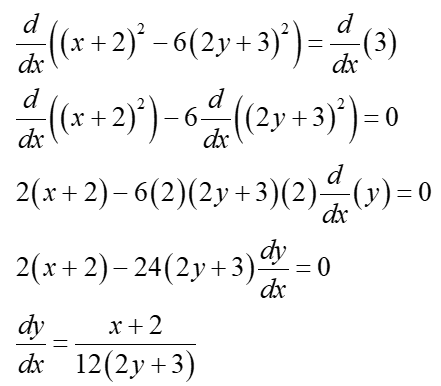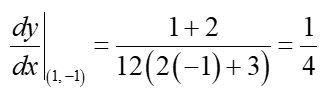# Find an equation of the tangent line at the given point.(x + 2)2 − 6(2y + 3)2 = 3, (1,−1)

Question
8 views

Find an equation of the tangent line at the given point.

(x + 2)2 − 6(2y + 3)2 = 3, (1,−1)

check_circle

Step 1

Consider

(x + 2)2 − 6(2y + 3)2 = 3, (1,−1)

Step 2

Find the slope of the equation using implicit differentiationStep 3

Find the slope at the point (1,−1)...

### Want to see the full answer?

See Solution

#### Want to see this answer and more?

Solutions are written by subject experts who are available 24/7. Questions are typically answered within 1 hour.*

See Solution
*Response times may vary by subject and question.
Tagged in